In :
from IPython.display import HTML

HTML('''<script>
code_show=true;
function code_toggle() {
if (code_show){
$('div.input').hide(); } else {$('div.input').show();
}
code_show = !code_show
}
$( document ).ready(code_toggle); </script> <form action="javascript:code_toggle()"><input type="submit" value="Click here to toggle on/off the raw code."></form>''')  Out: # Selection process for CS50 Puyo¶ This notebook contains information about the selection process applied to the candidates of CS50 Puyo. The process started with 18 candidates competing for 8 available seats. The candidates took a test and were interviewed by the instructor of the course. In the end, 4 men and 4 women with the highest scores were chosen. ## Test¶ The test consisted of 20 questions, 17 questions about mathematical problems and 3 open-ended questions. The math section was graded over 82 points and the questions section over 18 points. ### Math Section¶ The average grade in this section is 12.8 points. Because this section is graded over 82, most scores are significantly low. There are 9 students with scores above the average. In : %matplotlib inline import pandas as pd import matplotlib.pyplot as plt c_orange = (255/255, 128/255, 14/255) student_numbers = list(range(19)) data = pd.read_csv('Applicants.csv', encoding='ansi') math_scores = data['Math (82)'] fig, ax = plt.subplots(figsize=(6,6)) ax.bar(data.index,math_scores) ax.axhline(math_scores.mean(), color=c_orange) ax.text(15.8, 14, 'Average', size=9.5) ax.axhline(82, color=c_orange) ax.text(12.8, 79, 'Max possible score', size=9.5) ax.set_title('Scores in math section', size=14, weight='bold') ax.tick_params(bottom='off', labelbottom='off', left='off') ax.spines['right'].set_visible(False) ax.spines['top'].set_visible(False) ax.spines['bottom'].set_visible(False) ax.spines['left'].set_visible(False) ax.set_ylim(0, 85) ax.set_xlabel('Student') plt.show()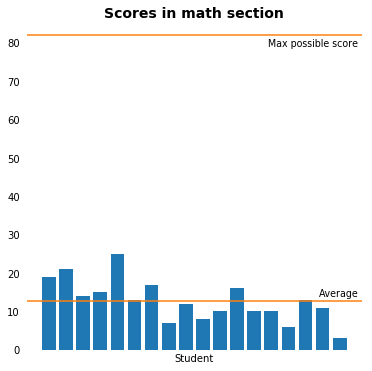### Questions Section¶ This section comprised 3 questions. The criteria for grading these questions were critical thinking and creativity. In : data_questions = [] data_questions.append(data['Internet (4)']) data_questions.append(data['Principal (7)']) data_questions.append(data['Crisis (7)']) questions = ['How would you explain the internet\nto a 3 year old?\n4 points', 'What three things would you change/enhance/implement\nat your high school if you were the principal?\n7 points', 'How could you solve humankind’s biggest crisis\ngiven$1 billion and a spacecraft?\n7 points']
fig = plt.figure(figsize=(20,6))
for i in range(3):
ax.set_title(questions[i])
ax.bar(data.index, data_questions[i])
ax.tick_params(bottom='off', labelbottom='off', left='off')
ax.spines['right'].set_visible(False)
ax.spines['top'].set_visible(False)
ax.spines['bottom'].set_visible(False)
ax.spines['left'].set_visible(False)
ax.axhline(data_questions[i].mean(), color=c_orange)
plt.show()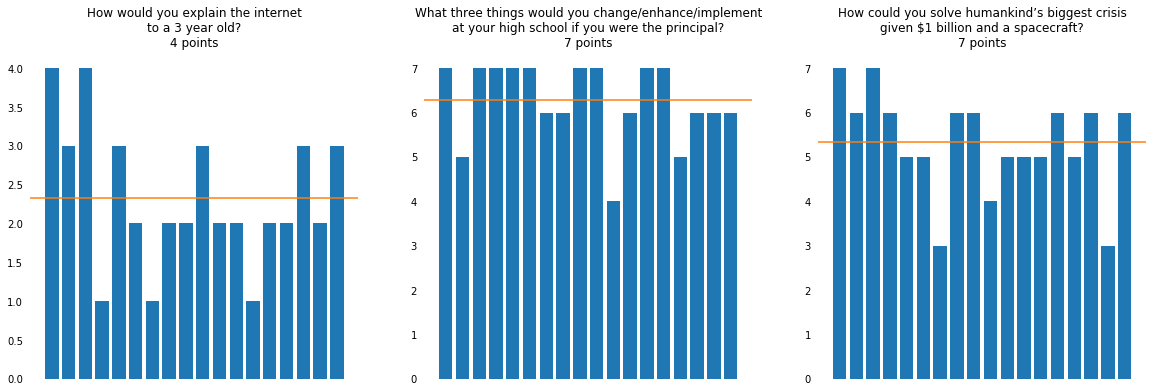## Total Scores in Test¶

The candidates are from the 1st grade and 2nd grade of high school. To compensate for this difference, a weight is given such that the candidates of 1st grade receive a 15% increase in their total score.

The highest total score is 42.6 points and the average is 28.4 points.

In :
fig, ax = plt.subplots(figsize=(6,6))
data['Weighted Total Test'] = data['Total'] * data['Weight']
total_test = data['Weighted Total Test']
ax.bar(data.index,total_test)
ax.axhline(total_test.mean(), color=c_orange)
ax.text(15.8, 30, 'Average', size=9.5)
ax.set_title('Total Scores in Test\nMath section + open-ended questions', size=15, weight='bold')
ax.tick_params(bottom='off', labelbottom='off', left='off')
ax.spines['right'].set_visible(False)
ax.spines['top'].set_visible(False)
ax.spines['bottom'].set_visible(False)
ax.spines['left'].set_visible(False)
ax.set_xlabel('Student')
ax.set_ylim(0, 100)
plt.show()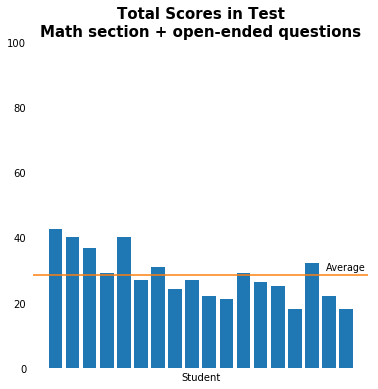## Interview¶

The personal interview comprised 10 questions and lasted 30 minutes. Six questions were the same for all candidates and four questions were selected randomly from a pool. The criteria for grading the interview were structure, argumentation, and use of examples.

In :
c_green = (63/255,124/255,123/255)
c_purple = (130/255,32/255,74/255)
data_interview = []
data_interview.append(data['Structure (50)'])
data_interview.append(data['Argumentative (20)'])
data_interview.append(data['Examples (30)'])
criteria = ['Structure\n50 points',
'Argumentation\n20 points',
'Examples\n30 points']
fig = plt.figure(figsize=(20,6))
for i in range(0,3):
ax.set_title(criteria[i])
ax.bar(data.index, data_interview[i], color=c_green)
ax.tick_params(bottom='off', labelbottom='off', left='off')
ax.spines['right'].set_visible(False)
ax.spines['top'].set_visible(False)
ax.spines['bottom'].set_visible(False)
ax.spines['left'].set_visible(False)
ax.axhline(data_interview[i].mean(), color=c_purple)
plt.show()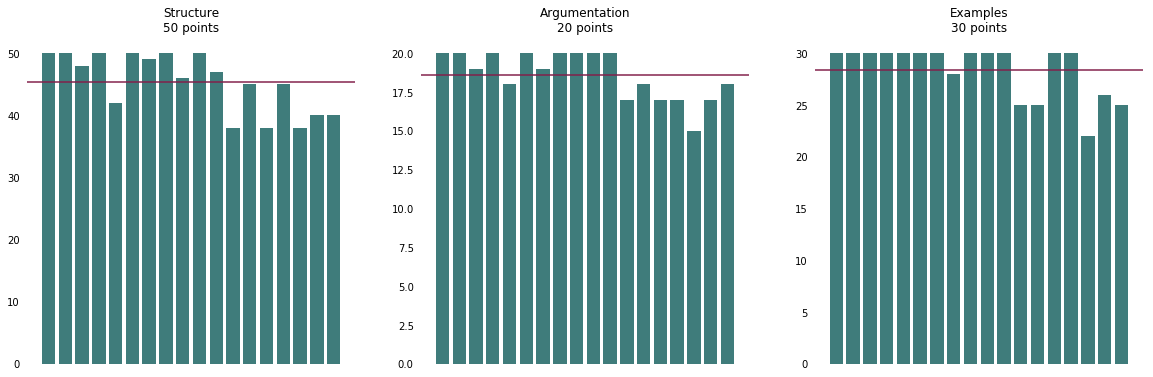## Total Scores in Interview¶

All candidates did well in the interview, the average score is 92.3 points.

In :
fig, ax = plt.subplots(figsize=(6,6))
total_interview = data['Total.1']
ax.bar(data.index,total_interview, color=c_green)
ax.axhline(total_interview.mean(), color=c_purple)
ax.text(15.8, 94, 'Average', size=9.5)
ax.set_title('Total Scores in Interview', size=15, weight='bold')
ax.tick_params(bottom='off', labelbottom='off', left='off')
ax.spines['right'].set_visible(False)
ax.spines['top'].set_visible(False)
ax.spines['bottom'].set_visible(False)
ax.spines['left'].set_visible(False)
ax.set_xlabel('Student')
plt.show()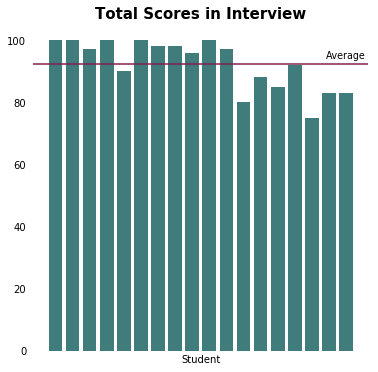## Personal traits¶

Based on the interview, the following traits were evaluated in the candidates and given a weight of 5 points.

• Curious
• Responsible
• Dedicated
• Organized
• Patient
• Hard-working
• Honest
In :
fig, ax = plt.subplots()
ax.bar(data.index, data['Traits'])
ax.set_title('Personal traits\n5 points', size=15, weight='bold')
ax.tick_params(bottom='on', labelbottom='on', left='off')
ax.spines['right'].set_visible(False)
ax.spines['top'].set_visible(False)
#ax.spines['bottom'].set_visible(False)
ax.spines['left'].set_visible(False)
ax.set_xlabel('Student')
ax.set_xticks(student_numbers)
plt.show()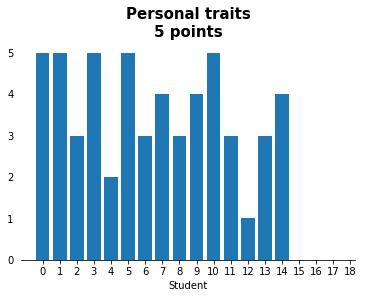## Results¶

The final scores are calculated in the following way:

• 60% test score
• 35% interview score
• 5% personal traits score
In :
fig, ax = plt.subplots(figsize=(6,6))
total = data['Total Results'] = (data['Weighted Total Test'] * 0.6) + (data['Total.1'] * 0.35) + (data['Traits'] * 0.05)
ax.bar(data.index,total, color='magenta')
#ax.axhline(total.mean(), color='black')
ax.set_title('FINAL SCORES', size=16, weight='bold')
ax.tick_params(bottom='off', labelbottom='off', left='off')
ax.spines['right'].set_visible(False)
ax.spines['top'].set_visible(False)
ax.spines['bottom'].set_visible(False)
ax.spines['left'].set_visible(False)
ax.set_xlabel('Student')
ax.set_ylim(0,100)
plt.show()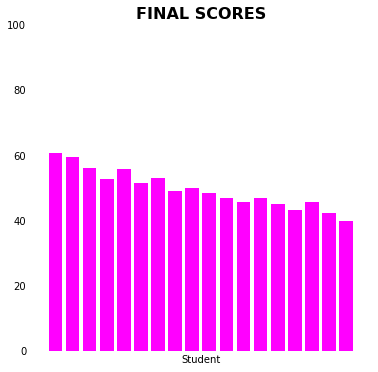## The selected students are:¶

• Brigitte Pérez
• Sebastián Illicachi
• Dayra Yasacama
• Daniel Velin
• Christian Santamaría
• Edwin León
In :
sorted_data = data.sort_values('Total Results', ascending=False)
all_gender = sorted_data['Gender']
names_final = pd.concat([female_final, male_final])
all_selected = sorted_data.loc[names_final.index]
#print(all_selected.sort_values('Total Results', ascending=False)['Name'])


### Personality Types¶

In :
from collections import Counter
personalities_count = Counter(data['Personality'])
df = pd.DataFrame.from_dict(personalities_count, orient='index')
df.plot(kind='bar', legend=False, title='Distribution of personality types\nAll applicants', rot=0)

personalities_selected_count = Counter(all_selected['Personality'])
df = pd.DataFrame.from_dict(personalities_selected_count, orient='index')
df.plot(kind='bar', legend=False, title='Distribution of personality types\nSucessfull applicants', rot=0)

Out:
<matplotlib.axes._subplots.AxesSubplot at 0x1a7a5b28208>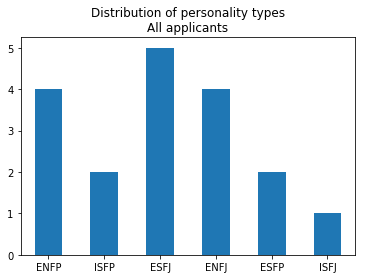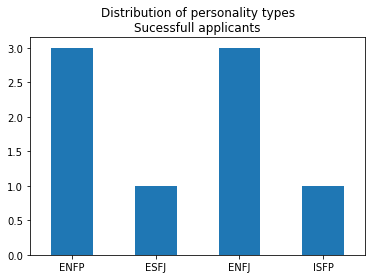### Course and Gender Average Scores¶

Female 52.9 45.9 49
Male 53 48.5 50
All 53 47.3 49.5
In :
data['Course'] = data['Class'].str.slice(0,1)
all_first_course = data[data['Course'] == '1']
all_second_course = data[data['Course'] == '2']
# print(data['Total Results'].mean())
# print(all_first_course['Total Results'].mean())
# print(all_second_course['Total Results'].mean())
# print(data[data['Gender'] == 'F']['Total Results'].mean())
# print(data[data['Gender'] == 'M']['Total Results'].mean())
# print(all_first_course[all_first_course['Gender'] == 'F']['Total Results'].mean())
# print(all_first_course[all_first_course['Gender'] == 'M']['Total Results'].mean())
# print(all_second_course[all_second_course['Gender'] == 'F']['Total Results'].mean())
# print(all_second_course[all_second_course['Gender'] == 'M']['Total Results'].mean())